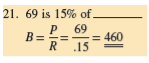• FAQ
• Contact/ Homework Answers / Mathematics / Solve the following for the base. R ound to hundredths
Not my Question
Flag Content

# Question : Solve the following for the base. R ound to hundredths

Solve the following for the base. R ound to hundredths when necessary.22. 360 is 150% of___________

23. 6.45 is 181/2% of____________

24. What is the base if the rate is 16.8% and the portion is 451?

25. What is the base if the portion is 10 and the rate is 2 3/4%?

26.

 What is the base if the portion is \$4,530 and the rate is 35%? Portion = Rate = Base =

## Solution 5 (1 Ratings )

Solved
Mathematics 1 Week Ago 8 Views